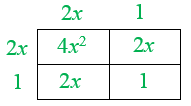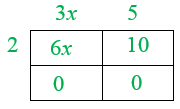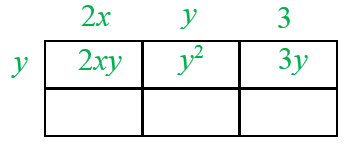Home > INT2 > Chapter 2 > Lesson 2.3.4 > Problem2-119

2-119.

Sketch an area model with the following dimensions on your paper, label the dimensions, and write an equivalence statement for its area as a product and as a sum. Homework Help ✎

 a. (2x + 1)(2x + 1)Hint (a):Remember to write each term in the expression outside of the boxes, and then multiply.Answer (a):Equivalence statement: $4x² + 4x + 1$ b. (2x)(4x)Hint (b):Refer to hint (a) when drawing the area model. c. 2(3x + 5)Answer (c):6x + 10d. y(2x + y + 3)Answer (d):$2xy + y^2 + 3y$